Published: 15 May 2017

# Vibration model of a multi-supported guide bar and analysis on the effect of supports location

Liuyuan Su1
Zhuo Meng2
Yujing Zhang3
Yize Sun4
1, 2, 3, 4College of Mechanical Engineering, Donghua University, Shanghai, China
Corresponding Author:
Zhuo Meng
Views 54

#### Abstract

Two methods (equivalent force method and segmental mode assuming method) of calculating the natural frequencies and mode shapes of a free-free-multi-supported beam subjected to an axial load is found, considering the structure characteristic of the guide bar, which has long length but small section, and supported by many bearings. The calculation shows that these two methods are convenient for computer programing and have the same results in obtaining the natural frequencies and mode shapes of a free-free-multi-supported beam subjected to an axial load, solving the problem that the vibration function of this kind of beam is hard to deal with because it cannot be simplified with the boundary condition of two ends. Then the segmental mode assuming method is used to analyze the impact of the support location on the natural frequencies and mode shapes of the guide bar. The relation graphs of the natural frequencies with support location, as well as the support locations where the natural frequencies reached the maximum and the minimum are found, providing a reference for the support location selection for the guide bar. The changing curves of the mode shapes with support location are plotted, which show that the bending deformation is homogeneous when the length of each segment is approximately equal, avoiding the phenomenon that bending stresses concentrates at the large-amplitude segments and cause breakage while less stress exists in small-amplitude segments and hinder the exploiting of their performance, providing a reference for the structure design of the guide bar.

## 1. Introduction

The guide bar shogging system was composited of servo motor, ball screw, spherical hinge, steel wire rope, guide bar and supporting elements, as showed in Fig. 1. Servo motor drives the ball screw, pushes out the guide bar through spherical hinge and hauls it back through steel wire rope. Guide bar with long length but small section, supported by many bearings, will vibrate under high speed movement, which influents the movement smoothness, causes transverse error, leads to “needle rubbing” phenomenon, and hinders the high speed development of warp knitting machine [1, 2].

Fig. 1The structure of the guide bar shogging system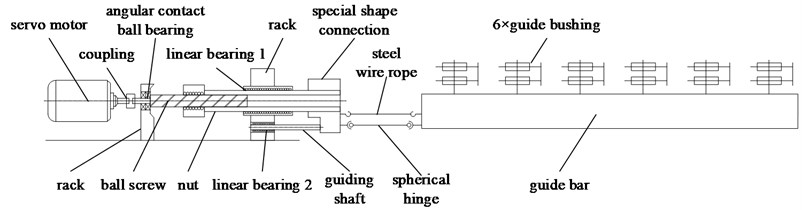After analyzing the model of guide bar, as showed in Fig. 2, it can be known that the guide bar satisfies the following conditions:

(1) The length of each segment (0.6 m) is more than 10 times the height of section (0.055 m).

(2) The length of a guide bushing (0.044 m) is much less than that of the guide bar (3.6 m).

(3) The transverse displacement (4×1.0583 = 4.2332 mm) can be ignored compared with the length of guide bar.

Therefore, guide bar can be considered as a free-free-multi-supported continuous beam subjected to an axial load, the main vibration form of which is bending vibration.

Fig. 2The model of guide bar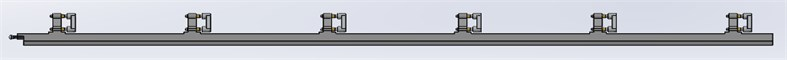Beam is widely used in the field of aviation, mechanism and architectural engineering. The vibration of multi-supported continuous beam is often approximately calculated by energy method, iteration method and transfer matrix method [1-24]. S.-W. Hong and J.-W. Kim applied Laplace transformation to a spatial state equation and came up with the dynamic matrix for the vibration analysis of multi-span Timoshenko beams . Yusuf Yesilce1et al. used secant method to obtain the frequencies values and mode shapes for the multi-span Timoshenko beam with multiple spring-mass systems . D. Y. Zheng et al. analyzed the vibration of a multi-span non-uniform beam subjected to a moving load based on Hamilton principle . Hai-Ping Lin et al. considered the compatibility requirements on each constraint point of Timoshenko beam and came up with the transfer matrix to deal with the free vibration analysis of a multi-span beam with an arbitrary number of flexible constraints . Hsien-Yuan Lin employed the numerical assembly method (NAM) to determine the “exact” frequency–response amplitudes of a multi-span beam carrying a number of various concentrated elements and subjected to a harmonic force . C. Johansson et al. applied the boundary conditions to the characteristic function of a beam and obtained its natural frequencies as well as its mode shapes, providing a closed-form solution for the vibration of continuous stepped beams under constant moving loads . M. Guebailia et al. solved the free vibration equation of a multi-span bridge deck by local estimation method, and estimated the coefficients at anti nodes of the mode shapes of the beam . D. ZHOU developed the static Timoshenko beam functions, which are composed of a set of transverse deflection functions and a set of rotational angle functions, and solved the vibration of a multi-span Timoshenko beam under a series of static sinusoidal loads distributed along the length of the beam . H. P. Lee analyzed the dynamic response of a multi-span beam on one-sided point constraints subjected to a moving load by Hamilton’s principle and the assumed mode method . WU Chongjian et al. used WPA method to obtain the dynamic response, modal shape and stress distribution of a multi-supported mast of a submarine .

However, most of the algorithms listed above aim at solving the vibration of hinged-hinged-multi-supported beam, and few of them involve the vibration calculation of a free-free-multi-supported beam. Also, some of the methods are too difficult for computers to calculate programmatically. Cause the displacement of two ends of a free-free-multi- supported beam isn’t fixed, the coordinate building method, as well as the vibration calculation method, is different with others. It is difficult to obtain the natural frequencies and mode shapes of a free-free-multi-supported beam because the vibration function of it cannot be simplified with the boundary condition of two ends as that of a hinged- hinged-multi-supported beam can. Two methods (equivalent force method and segmental mode assuming method) of obtaining the natural frequencies and the mode shapes of a free-free-multi-supported beam are presented based on the vibration analysis of a guide bar. The analysis shows that these two methods are convenient for computer programing and have the same results in obtaining the natural frequencies and mode shapes of a free-free-multi-supported beam subjected to an axial load. Later, the segmental mode assuming method is used to analyze the effect of supports location on frequencies and the mode shapes of the guide bar.

## 2. Equivalent force method

The method which replaces $n$ supports of a free-free-multi-supported beam subjected to an axial load by support constrained forces ${r}_{i}\left(t\right)$ ($i=$1, 2,…, $n$), transforming into the problem of solving the vibration of a free-free beam subjected to an axial load and $n$ support constrained forces, is named Equivalent force method . The coordinate origin point of the model should be put on the place of the first support, making the coordinate fixed, as showed in Fig. 3. The frequency equation and mode function can be derived through the vibration differential equation and constraint conditions of the beam based on this model.

Fig. 3The model of the equivalent force method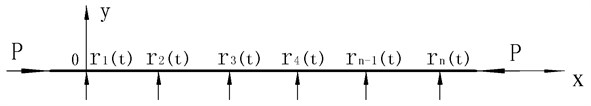### 2.1. Vibration differential equation and constraint conditions in equivalent force method

The vibration differential equation of a beam subjected to an axial load is as below:

1
$EI\left(\frac{{\partial }^{4}y}{\partial {x}^{4}}\right)+P\left(\frac{{\partial }^{2}y}{\partial {x}^{2}}\right)+\rho A\left(\frac{{\partial }^{2}y}{\partial {t}^{2}}\right)=\sum _{i=1}^{n}{r}_{i}\left(t\right)\delta \left(x-{x}_{i}\right),$

where $\delta \left(x-{x}_{i}\right)$ is the impulse function. $EI$ is the flexural rigidity, $A$ is the cross section area, $L$ is the length, and $\rho$ is the density of the beam. The location coordinate of the support is ${x}_{i}$ ($i=$1, 2,…, $n$) $\left(-{L}_{1}<{x}_{i}.

The bending moment as well as the shear force of the beam’s free ends is 0, so the boundary conditions [15, 18] are:

2
$\left\{\begin{array}{l}{\frac{{\partial }^{2}y\left(x,t\right)}{\partial {x}^{2}}|}_{x=-{L}_{1}}={\frac{{\partial }^{2}y\left(x,t\right)}{\partial {x}^{2}}|}_{x=L-{L}_{1}}=0,\\ {\frac{{\partial }^{3}y\left(x,t\right)}{\partial {x}^{3}}|}_{x=-{L}_{1}}={\frac{{\partial }^{3}y\left(x,t\right)}{\partial {x}^{3}}|}_{x=L-{L}_{1}}=0.\end{array}\right\$

The displacement constraint condition:

3
$y\left({x}_{i},t\right)=0,i=1,2,\dots ,n.$

### 2.2. The frequency equation and mode function in equivalent force method

The laws of the support constrained forces is the same as that of the free vibration of a beam, so let $y\left(x,t\right)=Y\left(x\right)\mathrm{s}\mathrm{i}\mathrm{n}\left(wt+\phi \right)$, ${r}_{i}\left(t\right)={R}_{i}\mathrm{s}\mathrm{i}\mathrm{n}\left(wt+\phi \right)$ ($i=$ 1, 2,…, $n$), put into Eqs. (1) and (2) and get:

${Y}^{\left(4\right)}\left(x\right)+{\alpha }^{2}{Y}^{\left(2\right)}\left(x\right)-{k}^{4}Y\left(x\right)=\sum _{i=1}^{n}\frac{{R}_{i}}{EI}\delta \left(x-{x}_{i}\right),$

where:

4
${\alpha }^{2}=\frac{P}{EI},{k}^{4}=\frac{\rho A{w}^{2}}{EI},$
5
${Y}^{\left(2\right)}\left(-{L}_{1}\right)={Y}^{\left(2\right)}\left(L-{L}_{1}\right)=0,$
6
${Y}^{\left(3\right)}\left(-{L}_{1}\right)={Y}^{\left(3\right)}\left(L-{L}_{1}\right)=0,$
7
$Y\left({x}_{i}\right)=0,i=1,2,\dots ,n.$

Implement the Laplace transformation on Eq. (4) and get:

8
${S}^{4}L\left(Y\right)-{S}^{3}Y\left(0\right)-{S}^{2}Y\text{'}\left(0\right)-S{Y}^{2}\left(0\right)-{Y}^{3}\left(0\right)+{\alpha }^{2}\left[{S}^{2}L\left(Y\right)-SY\left(0\right)-Y\text{'}\left(0\right)\right]$
$-{k}^{4}L\left(Y\right)=\sum _{i=1}^{n}\frac{{R}_{i}}{EI}{e}^{-S{x}_{i}}.$

Namely:

9
$L\left(Y\right)=\frac{1}{\left({S}^{2}+{{\lambda }_{1}}^{2}\right)\left({S}^{2}-{{\lambda }_{2}}^{2}\right)}\left[\begin{array}{c}{Y}^{3}\left(0\right)+{\alpha }^{2}{Y}^{\text{'}}\left(0\right)+S\left[{Y}^{2}\left(0\right)+{\alpha }^{2}Y\left(0\right)\right]\\ +{S}^{2}{Y}^{\text{'}}\left(0\right)+{S}^{3}Y\left(0\right)+\sum _{i=1}^{n}\frac{{R}_{i}}{EI}{e}^{-S{x}_{i}}\end{array}\right],$

where:

10
$\left\{\begin{array}{l}{\lambda }_{1}=\sqrt{\frac{{\alpha }^{2}}{2}+\sqrt{{k}^{4}+\frac{{\alpha }^{4}}{4}}},\\ {\lambda }_{2}=\sqrt{-\frac{{\alpha }^{2}}{2}+\sqrt{{k}^{4}+\frac{{\alpha }^{4}}{4}.}}\end{array}\right\$

Implement the inverse Laplace transformation on Eq. (9) and get:

11
$Y\left(x\right)={D}_{1}\mathrm{s}\mathrm{i}\mathrm{n}{\lambda }_{1}x+{D}_{2}\mathrm{c}\mathrm{o}\mathrm{s}{\lambda }_{1}x+{D}_{3}\mathrm{s}\mathrm{i}\mathrm{n}\mathrm{h}{\lambda }_{2}x+{D}_{4}\mathrm{c}\mathrm{o}\mathrm{s}\mathrm{h}{\lambda }_{2}x$
$+\frac{1}{{{\lambda }_{1}}^{2}+{{\lambda }_{2}}^{2}}\sum _{i=1}^{n}\frac{{R}_{i}}{EI}\left[\frac{1}{{\lambda }_{2}}\mathrm{sinh}{\lambda }_{2}\left(x-{x}_{i}\right)-\frac{1}{{\lambda }_{1}}\mathrm{sin}\left(\mathrm{x}-{x}_{i}\right)\right]u\left(x-{x}_{i}\right),$

where ${D}_{1}$, ${D}_{2}$, ${D}_{3}$, ${D}_{4}$ are the undetermined coefficients, which are determined by the boundary conditions, $u\left(x-{x}_{i}\right)$ is the unit step function, namely:

$u\left(x-{x}_{i}\right)=\left\{\begin{array}{l}0,x<{x}_{i},\\ 1,x>{x}_{i},\end{array}\right\i=1,2,\dots ,n.$

Take a derivative of the formula above and get:

12
$\left\{\begin{array}{l}-{{\lambda }_{1}}^{2}{D}_{1}\mathrm{s}\mathrm{i}\mathrm{n}{\lambda }_{1}\left(-{L}_{1}\right)-{{\lambda }_{1}}^{2}{D}_{2}\mathrm{c}\mathrm{o}\mathrm{s}{\lambda }_{1}\left(-{L}_{1}\right)+{{\lambda }_{2}}^{2}{D}_{3}\mathrm{s}\mathrm{i}\mathrm{n}\mathrm{h}{\lambda }_{2}\left(-{L}_{1}\right)\\ +{{\lambda }_{2}}^{2}{D}_{4}\mathrm{c}\mathrm{o}\mathrm{s}\mathrm{h}{\lambda }_{2}\left(-{L}_{1}\right)=0,\\ -{{\lambda }_{1}}^{3}{D}_{1}\mathrm{c}\mathrm{o}\mathrm{s}{\lambda }_{1}\left(-{L}_{1}\right)+{{\lambda }_{1}}^{3}{D}_{2}\mathrm{s}\mathrm{i}\mathrm{n}{\lambda }_{1}\left(-{L}_{1}\right)+{{\lambda }_{2}}^{3}{D}_{3}\mathrm{c}\mathrm{o}\mathrm{s}\mathrm{h}{\lambda }_{2}\left(-{L}_{1}\right)\\ +{{\lambda }_{2}}^{3}{D}_{4}\mathrm{s}\mathrm{i}\mathrm{n}\mathrm{h}{\lambda }_{2}\left(-{L}_{1}\right)=0,\\ -{{\lambda }_{1}}^{2}{D}_{1}\mathrm{s}\mathrm{i}\mathrm{n}{\lambda }_{1}\left(L-{L}_{1}\right)-{{\lambda }_{1}}^{2}{D}_{2}\mathrm{c}\mathrm{o}\mathrm{s}{\lambda }_{1}\left(L-{L}_{1}\right)+{{\lambda }_{2}}^{2}{D}_{3}\mathrm{s}\mathrm{i}\mathrm{n}\mathrm{h}{\lambda }_{2}\left(L-{L}_{1}\right)\\ +{{\lambda }_{2}}^{2}{D}_{4}\mathrm{c}\mathrm{o}\mathrm{s}\mathrm{h}{\lambda }_{2}\left(L-{L}_{1}\right)+\frac{1}{{{\lambda }_{1}}^{2}+{{\lambda }_{2}}^{2}}\sum _{i=1}^{n}\frac{{R}_{i}}{EI}\left[\begin{array}{c}{\lambda }_{2}sinh{\lambda }_{2}\left(L-{L}_{1}-{x}_{i}\right)\\ +{\lambda }_{1}sin{\lambda }_{1}\left(L-{L}_{1}-{x}_{i}\right)\end{array}\right]=0,\\ -{{\lambda }_{1}}^{3}{D}_{1}\mathrm{c}\mathrm{o}\mathrm{s}{\lambda }_{1}\left(L-{L}_{1}\right)+{{\lambda }_{1}}^{3}{D}_{2}\mathrm{s}\mathrm{i}\mathrm{n}{\lambda }_{1}\left(L-{L}_{1}\right)+{{\lambda }_{2}}^{3}{D}_{3}\mathrm{c}\mathrm{o}\mathrm{s}\mathrm{h}{\lambda }_{2}\left(L-{L}_{1}\right)\\ +{{\lambda }_{2}}^{3}{D}_{4}\mathrm{s}\mathrm{i}\mathrm{n}\mathrm{h}{\lambda }_{2}\left(L-{L}_{1}\right)+\frac{1}{{{\lambda }_{1}}^{2}+{{\lambda }_{2}}^{2}}\sum _{i=1}^{n}\frac{{R}_{i}}{EI}\left[\begin{array}{c}{{\lambda }_{2}}^{2}cosh{\lambda }_{2}\left(L-{L}_{1}-{x}_{i}\right)\\ +{{\lambda }_{1}}^{2}cosh{\lambda }_{1}\left(L-{L}_{1}-{x}_{i}\right)\end{array}\right]=0.\end{array}\right\$

It can be known that ${D}_{1}$, ${D}_{2}$, ${D}_{3}$, ${D}_{4}$ are complex expressions containing variable ${x}_{i}$ and ${R}_{i}$ ($i=$ 1, 2,…, $n$), which result from the coupling of all support constrained forces. Because the coordinate origin point isn’t put on the end of the beam, Eq. (12) can’t be simplified by the boundary condition as in article , and unfit for computer programing. Symbol ${D}_{k}^{*}\left({x}_{i}\right)$ is introduced to decouple Eq. (12) based on its characteristics. ${D}_{k}^{*}\left({x}_{i}\right)$ is an equation containing only ${x}_{i}$ ($k=$ 1, 2, 3, 4; $i=$ 1, 2,… or $n$), based on which ${D}_{1}$, ${D}_{2}$, ${D}_{3}$, ${D}_{4}$ can be expressed as below:

13
${D}_{k}=\frac{1}{{{\lambda }_{1}}^{2}+{{\lambda }_{2}}^{2}}\sum _{i=1}^{n}\frac{{R}_{i}}{EI}{D}_{k}^{*}\left({x}_{i}\right),k=1,2,3,4,i=1,2,\dots ,n.$

Displacement function can be expressed as:

14
$Y\left(x\right)=\frac{1}{{{\lambda }_{1}}^{2}+{{\lambda }_{2}}^{2}}\sum _{i=1}^{n}\frac{{R}_{i}}{EI}\left\{{D}_{1}^{*}\left({x}_{i}\right)\mathrm{s}\mathrm{i}\mathrm{n}{\lambda }_{1}x+{D}_{2}^{*}\left({x}_{i}\right)\mathrm{c}\mathrm{o}\mathrm{s}{\lambda }_{1}x+{D}_{3}^{*}\left({x}_{i}\right)\mathrm{s}\mathrm{i}\mathrm{n}\mathrm{h}{\lambda }_{2}x\right\$
$+{D}_{4}^{*}\left({x}_{i}\right)\mathrm{c}\mathrm{o}\mathrm{s}\mathrm{h}{\lambda }_{2}x+\left[\frac{1}{{\lambda }_{2}}\mathrm{s}\mathrm{i}\mathrm{n}\mathrm{h}{\lambda }_{2}\left(x-{x}_{i}\right)-\frac{1}{{\lambda }_{1}}\mathrm{s}\mathrm{i}\mathrm{n}{\lambda }_{1}\left(x-{x}_{i}\right)\right]u\left(x-{x}_{i}\right)}.$

Substitute Eq. (11) into Eq. (7), and express the result as the form of matrix, then:

15
$\left[A\right]\left\{R\right\}=0,$

where $\left\{R\right\}={\left[{R}_{1},{R}_{2},{R}_{3},\dots ,{R}_{n}\right]}^{T}$. Because ${R}_{i}$ ($i=$ 1, 2, 3,…, $n$) are not all 0, the determinant of Eq. (12) should be 0, namely:

16
$\left|A\right|={\left|{A}_{ji}\right|}_{n×n}=0,$

where:

17
${A}_{ji}=\frac{1}{\left({{\lambda }^{2}}_{1}+{{\lambda }^{2}}_{2}\right)EI}\left\{{D}_{1}^{*}\left({x}_{i}\right)\mathrm{s}\mathrm{i}\mathrm{n}{\lambda }_{1}{x}_{j}+{D}_{2}^{*}\left({x}_{i}\right)\mathrm{c}\mathrm{o}\mathrm{s}{\lambda }_{1}{x}_{j}+{D}_{3}^{*}\left({x}_{i}\right)\mathrm{s}\mathrm{i}\mathrm{n}\mathrm{h}{\lambda }_{2}{x}_{j}\right\$
$+{D}_{4}^{*}\left({x}_{i}\right)\mathrm{c}\mathrm{o}\mathrm{s}\mathrm{h}{\lambda }_{2}{x}_{j}+\left[\frac{1}{{\lambda }_{2}}\mathrm{s}\mathrm{i}\mathrm{n}\mathrm{h}{\lambda }_{2}\left({x}_{j}-{x}_{i}\right)-\frac{1}{{\lambda }_{1}}\mathrm{s}\mathrm{i}\mathrm{n}{\lambda }_{1}\left({x}_{j}-{x}_{i}\right)\right]u\left({x}_{j}-{x}_{i}\right)},$
$i,j=1,2,3,\dots ,n.$

According to the analysis above, in order to solve Eq. (16), the value of ${D}_{k}^{*}\left({x}_{i}\right)$ should be obtained ($k=$ 1, 2, 3, 4; $i=$ 1, 2,…, $n$), which only need to satisfy Eq. (18). The equation is simplified and fit for computer programing:

18
$\left\{\begin{array}{c}\begin{array}{l}-{\lambda }_{1}^{2}{D}_{1}^{*}\left({x}_{i}\right)\mathrm{s}\mathrm{i}\mathrm{n}{\lambda }_{1}\left(-{L}_{1}\right)-{\lambda }_{1}^{2}{D}_{2}^{*}\left({x}_{i}\right)\mathrm{c}\mathrm{o}\mathrm{s}{\lambda }_{1}\left(-{L}_{1}\right)+{\lambda }_{2}^{2}{D}_{3}^{*}\left({x}_{i}\right)\mathrm{s}\mathrm{i}\mathrm{n}\mathrm{h}{\lambda }_{2}\left(-{L}_{1}\right)\\ +{\lambda }_{2}^{2}{D}_{4}^{*}\left({x}_{i}\right)\mathrm{c}\mathrm{o}\mathrm{s}\mathrm{h}{\lambda }_{2}\left(-{L}_{1}\right)=0,\\ -{\lambda }_{1}^{3}{D}_{1}^{*}\left({x}_{i}\right)\mathrm{c}\mathrm{o}\mathrm{s}{\lambda }_{1}\left(-{L}_{1}\right)+{\lambda }_{1}^{3}{D}_{2}^{*}\left({x}_{i}\right)\mathrm{s}\mathrm{i}\mathrm{n}{\lambda }_{1}\left(-{L}_{1}\right)+{\lambda }_{2}^{3}{D}_{3}^{*}\left({x}_{i}\right)\mathrm{c}\mathrm{o}\mathrm{s}\mathrm{h}{\lambda }_{2}\left(-{L}_{1}\right)\\ +{\lambda }_{2}^{3}{D}_{4}^{*}\left({x}_{i}\right)\mathrm{s}\mathrm{i}\mathrm{n}\mathrm{h}{\lambda }_{2}\left(-{L}_{1}\right)=0,\\ -{\lambda }_{1}^{2}{D}_{1}^{*}\left({x}_{i}\right)\mathrm{s}\mathrm{i}\mathrm{n}{\lambda }_{1}\left(L-{L}_{1}\right)-{\lambda }_{1}^{2}{D}_{2}^{*}\left({x}_{i}\right)\mathrm{c}\mathrm{o}\mathrm{s}{\lambda }_{1}\left(L-{L}_{1}\right)+{\lambda }_{2}^{2}{D}_{3}^{*}\left({x}_{i}\right)\mathrm{s}\mathrm{i}\mathrm{n}\mathrm{h}{\lambda }_{2}\left(L-{L}_{1}\right)\\ +{\lambda }_{2}^{2}{D}_{4}^{*}\left({x}_{i}\right)\mathrm{h}{\lambda }_{2}\left(L-{L}_{1}\right)+\mathrm{c}\mathrm{o}\mathrm{s}\left[{\lambda }_{2}\mathrm{s}\mathrm{i}\mathrm{n}\mathrm{h}{\lambda }_{2}\left(L-{L}_{1}-{x}_{i}\right)+{\lambda }_{1}\mathrm{s}\mathrm{i}\mathrm{n}{\lambda }_{1}\left(L-{L}_{1}-{x}_{i}\right)\right]=0,\\ -{\lambda }_{1}^{3}{D}_{1}^{*}\left({x}_{i}\right)\mathrm{c}\mathrm{o}\mathrm{s}{\lambda }_{1}\left(L-{L}_{1}\right)+{\lambda }_{1}^{3}{D}_{2}^{*}\left({x}_{i}\right)\mathrm{s}\mathrm{i}\mathrm{n}{\lambda }_{1}\left(L-{L}_{1}\right)+{\lambda }_{2}^{3}{D}_{3}^{*}\left({x}_{i}\right)\mathrm{c}\mathrm{o}\mathrm{s}\mathrm{h}{\lambda }_{2}\left(L-{L}_{1}\right)\\ +{\lambda }_{2}^{3}{D}_{4}^{*}\left({x}_{i}\right){\lambda }_{2}\left(L-{L}_{1}\right)+\mathrm{s}\mathrm{i}\mathrm{n}\mathrm{h}\left[{\lambda }_{2}^{2}\mathrm{c}\mathrm{o}\mathrm{s}h{\lambda }_{2}\left(L-{L}_{1}-{x}_{i}\right)+{\lambda }_{1}^{3}\mathrm{c}\mathrm{o}\mathrm{s}{\lambda }_{1}\left(L-{L}_{1}-{x}_{i}\right)\right]=0,\end{array}\end{array}\right\$
$i=1,2,\dots ,n.$

When there are plenty of supports and the higher-order determinant is hard to solve, the converse numerical solution method can be used. Firstly, determine the range and the gradient of value $w$. Secondly, combine ${\alpha }^{2}=P/EI$, ${k}^{4}=\rho A{w}^{2}/EI$, and Eq. (10) to calculate the corresponding ${\lambda }_{1}$ and ${\lambda }_{2}$, and then use Eq. (18) to obtain the value of corresponding ${D}_{k}^{*}\left({x}_{i}\right)$ ($k=$ 1, 2, 3, 4; $i=$1, 2,…or $n$). Thirdly, put them into Eq. (16) and judge whether they are the roots of the equation set. Fourthly, put the satisfied value of $w$ into $f=w/2\pi$ and the natural frequency $f$ can be obtained. Finally, put the calculated $w$ and the corresponding ${\lambda }_{1}$, ${\lambda }_{2}$, and ${D}_{k}^{*}\left({x}_{i}\right)$ into Eq. (14) and the mode shapes of the free-free-multi-supported beam can be obtained. Pay attention to the mode function Eq. (14), the second half of which are expressed by step function, and add judgement when programing.

## 3. The segmental mode assuming method

The method which divides the free-free beam with $n$ supports into $n+1$ segments at each support, assume the mode function of each segment and then create the frequency equation and mode function through boundary and connecting conditions, is called Equivalent force method. The coordinate origin point of the model should be put on the place of the first support, making the coordinate fixed, as showed in Fig. 4. The length of each segment is ${L}_{1}$, ${L}_{2}$, ${L}_{3}$,…, ${L}_{n+1}$.

Fig. 4The model of the segmental mode assuming method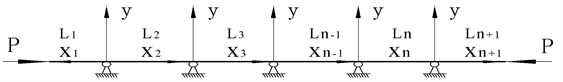### 3.1. Vibration differential equation and constraint conditions in segmental mode assuming method

According to the general solution of the free vibration of a beam , the mode function of each segment of the beam is assumed as:

19
${Y}_{i}\left({x}_{i}\right)={A}_{i}\mathrm{s}\mathrm{i}\mathrm{n}{\lambda }_{1}{x}_{i}+{B}_{i}\mathrm{c}\mathrm{o}\mathrm{s}{\lambda }_{1}{x}_{i}+{C}_{i}\mathrm{s}\mathrm{i}\mathrm{n}\mathrm{h}{\lambda }_{2}{x}_{i}+{D}_{i}\mathrm{c}\mathrm{o}\mathrm{s}\mathrm{h}{\lambda }_{2}{x}_{i},i=1,2,3,\dots ,n+1.$

The bending moment as well as the shear force of the beam’s free ends is 0, so the boundary conditions are as below:

20
$\left\{\begin{array}{l}{\frac{{\partial }^{2}{Y}_{1}}{\partial {{x}_{1}}^{2}}|}_{{x}_{1}={L}_{1}}=-{\lambda }_{1}^{2}{A}_{1}\mathrm{s}\mathrm{i}\mathrm{n}{\lambda }_{1}{L}_{1}-{\lambda }_{1}^{2}{B}_{1}\mathrm{c}\mathrm{o}\mathrm{s}{\lambda }_{1}{L}_{1}+{\lambda }_{2}^{2}{C}_{1}\mathrm{s}\mathrm{i}\mathrm{n}\mathrm{h}{\lambda }_{2}{L}_{1}+{\lambda }_{2}^{2}{D}_{1}\mathrm{c}\mathrm{o}\mathrm{s}\mathrm{h}{\lambda }_{2}{L}_{1}=0,\\ {\frac{{\partial }^{3}{Y}_{1}}{\partial {{x}_{1}}^{3}}|}_{{x}_{1}={L}_{1}}=-{\lambda }_{1}^{3}{A}_{1}\mathrm{c}\mathrm{o}\mathrm{s}{\lambda }_{1}{L}_{1}+{\lambda }_{1}^{3}{B}_{1}\mathrm{s}\mathrm{i}\mathrm{n}{\lambda }_{1}{L}_{1}+{\lambda }_{2}^{3}{C}_{1}\mathrm{c}\mathrm{o}\mathrm{s}\mathrm{h}{\lambda }_{2}{L}_{1}+{\lambda }_{2}^{3}{D}_{1}\mathrm{s}\mathrm{i}\mathrm{n}\mathrm{h}{\lambda }_{2}{L}_{1}=0,\\ {\frac{{\partial }^{2}{Y}_{n+1}}{\partial {{x}_{n}}^{2}}|}_{{x}_{n+1}={L}_{n+1}}=-{\lambda }_{1}^{2}{A}_{1}\mathrm{s}\mathrm{i}\mathrm{n}{\lambda }_{1}{L}_{n+1}-{\lambda }_{1}^{2}{B}_{1}\mathrm{c}\mathrm{o}\mathrm{s}{\lambda }_{1}{L}_{n+1}+{\lambda }_{2}^{2}{C}_{1}\mathrm{s}\mathrm{i}\mathrm{n}\mathrm{h}{\lambda }_{2}{L}_{n+1}\\ +{\lambda }_{2}^{2}{D}_{1}\mathrm{c}\mathrm{o}\mathrm{s}\mathrm{h}{\lambda }_{2}{L}_{n+1}=0,\\ {\frac{{\partial }^{2}{Y}_{n+1}}{\partial {{x}_{n}}^{2}}|}_{{x}_{n+1}={L}_{n+1}}=-{\lambda }_{1}^{3}{A}_{1}\mathrm{c}\mathrm{o}\mathrm{s}{\lambda }_{1}{L}_{n+1}+{\lambda }_{1}^{3}{B}_{1}\mathrm{s}\mathrm{i}\mathrm{n}{\lambda }_{1}{L}_{n+1}+{\lambda }_{2}^{3}{C}_{1}\mathrm{c}\mathrm{o}\mathrm{s}\mathrm{h}{\lambda }_{2}{L}_{n+1}\\ +{\lambda }_{2}^{3}{D}_{1}\mathrm{s}\mathrm{i}\mathrm{n}\mathrm{h}{\lambda }_{2}{L}_{n+1}=0.\end{array}\right\$

According to the continue conditions then:

21
$\left\{\begin{array}{l}{Y}_{1}\left(0\right)=0,\\ {Y}_{2}\left(0\right)=0,\\ {\frac{\partial {Y}_{1}}{\partial {x}_{1}}|}_{{x}_{1}=0}=-{\frac{\partial {Y}_{2}}{\partial {x}_{2}}|}_{{x}_{2}=0},\\ {\frac{{\partial }^{2}{Y}_{1}}{\partial {{x}_{1}}^{2}}|}_{{x}_{1}=0}={\frac{{\partial }^{2}{Y}_{2}}{\partial {{x}_{2}}^{2}}|}_{{x}_{2}=0},\\ {Y}_{i-1}\left({L}_{i-1}\right)=0,\\ {Y}_{i}\left(0\right)=0,\\ {\frac{\partial {Y}_{i-1}}{\partial {x}_{i-1}}|}_{{x}_{i-1}={{L}_{i-}}_{1}}={\frac{\partial {Y}_{i}}{\partial {x}_{i}}|}_{{x}_{i}=0},\\ {\frac{{\partial }^{2}{Y}_{i-1}}{\partial {{x}_{i-1}}^{2}}|}_{{x}_{i-1}={{L}_{i-}}_{1}}={\frac{{\partial }^{2}{Y}_{i}}{\partial {{x}_{i}}^{2}}|}_{{x}_{i}=0},i=3,4,5,\dots ,n+1.\end{array}\right\$

Put Eq. (19) into (21) and get the Eq. (22):

22
$\left\{\begin{array}{l}{B}_{1}+{D}_{1}=0,\\ {B}_{2}+{D}_{2}=0,\\ {\lambda }_{1}{A}_{1}+{\lambda }_{2}{C}_{2}=-\left({\lambda }_{1}{A}_{2}+{\lambda }_{2}{C}_{2}\right),\\ -{\lambda }_{1}^{2}{B}_{1}+{\lambda }_{2}^{2}{D}_{1}=-{\lambda }_{1}^{2}{B}_{2}+{\lambda }_{2}^{2}{D}_{2},\\ {A}_{i-1}\mathrm{s}\mathrm{i}\mathrm{n}{\lambda }_{1}{L}_{i-1}+{B}_{i-1}\mathrm{c}\mathrm{o}\mathrm{s}{\lambda }_{1}{L}_{i-1}+{C}_{i-1}\mathrm{s}\mathrm{i}\mathrm{n}\mathrm{h}{\lambda }_{2}{L}_{i-1}+{D}_{i-1}\mathrm{c}\mathrm{o}\mathrm{s}\mathrm{h}{\lambda }_{2}{L}_{i-1}=0,\\ {B}_{i}+{D}_{i}=0,\\ {\lambda }_{1}{A}_{i-1}\mathrm{c}\mathrm{o}\mathrm{s}{\lambda }_{1}{L}_{i-1}-{\lambda }_{1}{B}_{i-1}\mathrm{s}\mathrm{i}\mathrm{n}{\lambda }_{1}{L}_{i-1}+{\lambda }_{2}{C}_{i-1}\mathrm{c}\mathrm{o}\mathrm{s}\mathrm{h}{\lambda }_{2}{L}_{i-1}\\ +{\lambda }_{2}{D}_{i-1}\mathrm{s}\mathrm{i}\mathrm{n}\mathrm{h}{\lambda }_{2}{L}_{i-1}={\lambda }_{1}{A}_{i}+{\lambda }_{2}{C}_{i},\\ -{\lambda }_{1}^{2}{A}_{i-1}\mathrm{s}\mathrm{i}\mathrm{n}{\lambda }_{1}{L}_{i-1}-{\lambda }_{1}^{2}{B}_{i-1}\mathrm{c}\mathrm{o}\mathrm{s}{\lambda }_{1}{L}_{i-1}+{\lambda }_{2}^{2}{C}_{i-1}\mathrm{s}\mathrm{i}\mathrm{n}\mathrm{h}{\lambda }_{2}{L}_{i-1}\\ +{\lambda }_{2}^{2}{D}_{i-1}\mathrm{c}\mathrm{o}\mathrm{s}\mathrm{h}{\lambda }_{2}{L}_{i-1}=-{\lambda }_{1}^{2}{B}_{i}+{\lambda }_{2}^{2}{D}_{i},\end{array}\right\$
$i=3,4,5,\cdots ,n+1.$

### 3.2. The frequency equation and mode function in segmental mode assuming method

Combine Eq. (20) and (22) then:

23
$\left[T\right]\left\{A\right\}=0,$

where:

$\left\{A\right\}=\left[{A}_{1},{B}_{1},{C}_{1},{D}_{1},{A}_{2},{B}_{2},{C}_{2},{D}_{2},{A}_{3},{B}_{3},{C}_{3},{D}_{3},\dots ,{A}_{n+1},{B}_{n+1},{C}_{n+1},{D}_{n+1}\right].$

Cause the coefficients of each mode function ${A}_{1}$, ${B}_{1}$, ${C}_{1}$, ${D}_{1}$, ${A}_{2}$, ${B}_{2}$, ${C}_{2}$, ${D}_{2}$, ${A}_{3}$, ${B}_{3}$, ${C}_{3}$, ${D}_{3}$, …, ${A}_{n+1}$, ${B}_{n+1}$, ${C}_{n+1}$, ${D}_{n+1}$ is not all 0, the coefficient matrix of the system of simultaneous equations:

24
${\left|T\right|}_{4\left(n+1\right)×4\left(n+1\right)}=0.$

Eq. (24) is the frequency equation of the free-free-multi-supported beam subjected to an axial load, and the coefficient matrix $T$ should meet the laws as Eq. (25).

The symbol $T\left(a:b,c:d\right)$ means the submatrix extracted from the ath to bth row and the cth and dth column of matrix $T$:

25
$\left\{\begin{array}{l}T\left(1:2,1:4\right)=\left[\begin{array}{llll}-{\lambda }_{1}^{2}\mathrm{s}\mathrm{i}\mathrm{n}{\lambda }_{1}{L}_{1}& -{\lambda }_{1}^{2}\mathrm{c}\mathrm{o}\mathrm{s}{\lambda }_{1}{L}_{1}& {\lambda }_{2}^{2}\mathrm{s}\mathrm{i}\mathrm{n}\mathrm{h}{\lambda }_{2}{L}_{1}& {\lambda }_{2}^{2}\mathrm{c}\mathrm{o}\mathrm{s}\mathrm{h}{\lambda }_{2}{L}_{1}\\ -{\lambda }_{1}^{3}\mathrm{c}\mathrm{o}\mathrm{s}\mathrm{h}{\lambda }_{1}{L}_{1}& {\lambda }_{1}^{3}\mathrm{s}\mathrm{i}\mathrm{n}{\lambda }_{1}{L}_{1}& {\lambda }_{2}^{3}\mathrm{c}\mathrm{o}\mathrm{s}\mathrm{h}{\lambda }_{2}{L}_{1}& {\lambda }_{2}^{3}\mathrm{c}\mathrm{o}\mathrm{s}\mathrm{h}{\lambda }_{2}{L}_{1}\end{array}\right],\\ T\left(3:4,4n-3:4n\right)=\left[\begin{array}{llll}-{\lambda }_{1}^{2}\mathrm{s}\mathrm{i}\mathrm{n}{\lambda }_{1}{L}_{n}& -{\lambda }_{1}^{2}\mathrm{c}\mathrm{o}\mathrm{s}{\lambda }_{1}{L}_{n}& {\lambda }_{2}^{2}\mathrm{s}\mathrm{i}\mathrm{n}\mathrm{h}{\lambda }_{2}{L}_{n}& {\lambda }_{2}^{2}\mathrm{c}\mathrm{o}\mathrm{s}\mathrm{h}{\lambda }_{2}{L}_{n}\\ -{\lambda }_{1}^{3}\mathrm{c}\mathrm{o}\mathrm{s}{\lambda }_{1}{L}_{n}& {\lambda }_{1}^{3}\mathrm{s}\mathrm{i}\mathrm{n}{\lambda }_{1}{L}_{n}& {\lambda }_{2}^{3}\mathrm{c}\mathrm{o}\mathrm{s}\mathrm{h}{\lambda }_{2}{L}_{n}& {\lambda }_{2}^{3}\mathrm{s}\mathrm{i}\mathrm{n}\mathrm{h}{\lambda }_{2}{L}_{n}\end{array}\right],\\ T\left(5:8,1:8\right)=\left[\begin{array}{cccccccc}0& 1& 0& 1& 0& 0& 0& 0\\ 0& 0& 0& 0& 0& 1& 0& 1\\ {\lambda }_{1}& 0& {\lambda }_{2}& 0& {\lambda }_{1}& 0& {\lambda }_{2}& 0\\ 0& -{\lambda }_{1}^{2}& 0& {\lambda }_{2}^{2}& 0& {\lambda }_{1}^{2}& 0& -{\lambda }_{2}^{2}\end{array}\right],t=3,4,5,\dots ,n+1,\\ T\left(4t-3:4t,4t-7:4t\right)=\\ \left[\begin{array}{cccccccc}\mathrm{s}\mathrm{i}\mathrm{n}{\lambda }_{1}{L}_{t-1}& \mathrm{c}\mathrm{o}\mathrm{s}{\lambda }_{1}{L}_{t-1}& \mathrm{s}\mathrm{i}\mathrm{n}\mathrm{h}{\lambda }_{2}{L}_{t-1}& \mathrm{c}\mathrm{o}\mathrm{s}\mathrm{h}{\lambda }_{2}{L}_{t-1}& 0& 0& 0& 0\\ 0& 0& 0& 0& 0& 1& 0& 1\\ {\lambda }_{1}\mathrm{c}\mathrm{o}\mathrm{s}{\lambda }_{1}{L}_{t-1}& -{\lambda }_{1}\mathrm{s}\mathrm{i}\mathrm{n}{\lambda }_{1}{L}_{t-1}& {\lambda }_{2}\mathrm{c}\mathrm{o}\mathrm{s}\mathrm{h}{\lambda }_{2}{L}_{t-1}& {\lambda }_{2}\mathrm{s}\mathrm{i}\mathrm{n}\mathrm{h}{\lambda }_{2}{L}_{t-1}& -{\lambda }_{1}& 0& -{\lambda }_{2}& 0\\ -{\lambda }_{1}^{2}\mathrm{s}\mathrm{i}\mathrm{n}{\lambda }_{1}{L}_{t-1}& -{\lambda }_{1}^{2}\mathrm{c}\mathrm{o}\mathrm{s}{\lambda }_{1}{L}_{t-1}& {\lambda }_{2}^{2}\mathrm{s}\mathrm{i}\mathrm{n}\mathrm{h}{\lambda }_{2}{L}_{t-1}& {\lambda }_{2}^{2}\mathrm{c}\mathrm{o}\mathrm{s}\mathrm{h}{\lambda }_{2}{L}_{t-1}& 0& {\lambda }_{1}^{2}& 0& -{\lambda }_{2}^{2}\end{array}\right].\end{array}\right\$

When there are plenty of supports and the higher-order determinant is hard to solve, the converse numerical solution method can be used. Firstly, determine the range and the gradient of value $w$. Secondly, combine ${\alpha }^{2}=P/EI$, ${k}^{4}=\rho A{w}^{2}/EI$, and Eq. (10) to calculate the corresponding ${\lambda }_{1}$ and ${\lambda }_{2}$, and then use Eq. (25) and judge whether they are the roots of the equation set. Thridly, put the satisfied value of $w$ into $f=w/2\pi$ and the natural frequency $f$ can be obtained. Finally, put the calculated $w$ and the corresponding ${\lambda }_{1}$, ${\lambda }_{2}$, and ${D}_{k}^{*}\left({x}_{i}\right)$ into Eq. (19) and the mode shape of the free-free-multi-supported beam can be obtained. The mode function Eq. (19) is also a segmented function, which can be expressed by step function as Eq. (26):

26
$Y\left(x\right)={Y}_{1}\left(x\right)\left[u\left(x+{L}_{1}\right)-u\left(x\right)\right]+{Y}_{2}\left(x\right)\left[u\left(x\right)-u\left(x-{L}_{2}\right)\right]$
$+{Y}_{3}\left(x\right)\left[u\left(x-{L}_{2}\right)-u\left(x-{L}_{2}-{L}_{3}\right)\right]+\cdots$
$+{Y}_{n}\left(x\right)\left[u\left(x-{L}_{2}-{L}_{3}-\cdots -{L}_{n-1}\right)-u\left(x-{L}_{2}-{L}_{3}-\cdots -{L}_{n}\right)\right]$
$+{Y}_{n+1}\left(x\right)\left[u\left(x-{L}_{2}-{L}_{3}-\cdots -{L}_{n}\right)-u\left(x-{L}_{2}-{L}_{3}-\cdots -{L}_{n}-{L}_{n+1}\right)\right].$

## 4. Vibration of the guide bar

### 4.1. The structure parameter of guide bar

This article takes the guide bar of a homemade warp knitting machine as the prototype, and the structure parameter of it is as below.

(1) Guide bar is made of Magnalium, of which the density $\rho =$1800 Kg/m3, and the elasticity modulus $E=$ 45 GPa.

Table 1The first six mode shapes of the guide bar with two methods

 Equivalent force method Segmental mode assuming method The 1st mode shape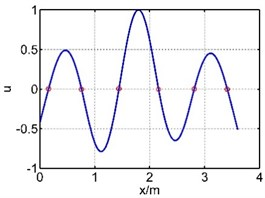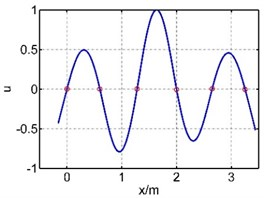The 2nd mode shape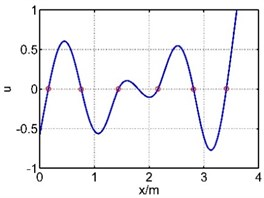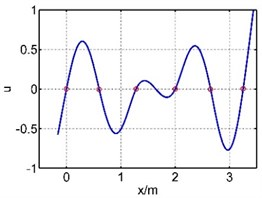The 3rd mode shape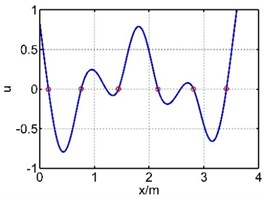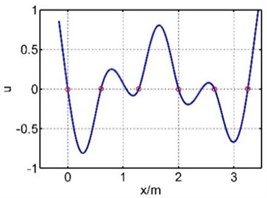The 4th mode shape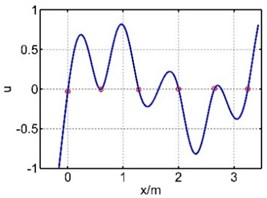The 5th mode shape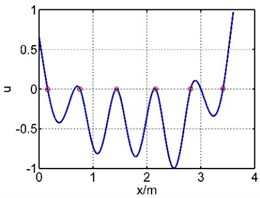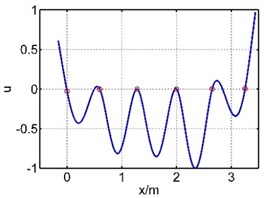The 6th mode shape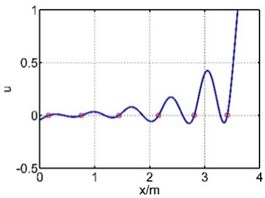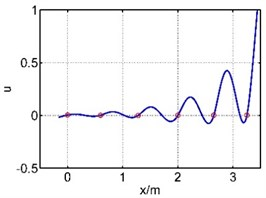(2) The length of the guide bar $L=$ 3.6 m. Take the end driven by spherical hinge as the beginning, then the length of each segment of the guide bar ${L}_{1}=$ 0.16 m, ${L}_{2}=$ 0.6 m, ${L}_{3}=$ 0.68 m, ${L}_{4}=$ 0.72 m, ${L}_{5}=$ 0.65 m, ${L}_{6}=$ 0.6 m, ${L}_{7}=$ 0.19 m. The axial load subjected by the guide bar $P=$ 5 N.

(3) The bending vibration of a guide bar will first occur in direction with the smallest cross sectional moment of inertia, and the calculated cross sectional moment of inertia ${I}_{y}=$ 4.11×10-8 m4.

### 4.2. The vibration of the guide bar with equivalent force method and segmental mode assuming method

The vibration of guide bar is analyzed by the equivalent force method and segmental mode assuming method. The first six mode shapes are showed in Table 1, where $x$ is the axial coordination of the guide bar defined by each method, and u is the amplitude (by normalization processing, taking the max amplitude as 1). It can be seen from Table 1 that the 1st mode shape consists of a single half wave in each span, and the amplitude of the mid span reaches the largest. The 2nd mode shape consists of two half waves in the mid span, while the 3th mode shape in the 3th and 5th spans, the 4th mode shape in the 4th and 6th spans, the 5th mode shape in the 2th and 6th spans, the 6th mode shape in each span. The mode shape is complex depending on the material properties and support location of the guide bar.

The first six natural frequencies calculated by these two methods are showed in Table 2. It can be seen from Table 2 that the relative error of natural frequencies calculated by these two methods are within 0.1 %, which depends mainly on the gradient of value $w$ when programing, so the results are consistent. It’s obvious that both these two methods do well in obtaining the natural frequency and mode shape of the multi-supported guide bar.

Table 2The first six natural frequencies of the guide bar with two methods

 Equivalent force method Segmental mode assuming method The 1st natural frequency / Hz 113.3183 113.2706 The 2nd natural frequency / Hz 135.2021 135.1958 The 3rd natural frequency / Hz 157.4042 157.4106 The 4th natural frequency / Hz 199.8986 200.2106 The 5th natural frequency / Hz 227.9099 227.9576 The 6th natural frequency / Hz 348.1514 348.1069

## 5. Analysis on the effect of support location

In the guide bar shogging system, guide bar with long length but small section, supported by many bearings, will vibrate under high speed movement, which influents the movement smoothness, causes transverse error, leads to “needle rubbing” phenomenon. Three methods are taken to increase the stiffness of the system for reducing vibration.

(1) Increase the cross-section area of the guide bar.

(2) Use high modulus and high elasticity materials.

(3) Optimize the support location of the guide bar.

Increasing the cross section area of the guide bar not only waste materials and make the system heavy, but also increase motor response time and energy consumption; Using high modulus and high elasticity materials (like Carbon fibre composite) to replace Magnalium materials not only make the production process complex but also increase the costs of the whole system; Optimizing the support location of the guide bar can reduce the vibration without adding materials, changing production process and increasing the costs. The impact of supports location on the natural frequencies and mode shapes will be studied later.

### 5.1. The impact of support location on natural frequencies

In the guide bar shogging system, the supports of guide bar are often arranged symmetrically for designing and assembling purposes. Use segmental mode assuming method to study the effect of the supports location and the constraint conditions are listed as below.

(1) Equality constraint:

27
$\left\{\begin{array}{l}{L}_{1}={L}_{7}=0.175\text{m},\\ {L}_{2}={L}_{6},\\ {L}_{3}={L}_{5},\\ \sum _{k=1}^{7}{L}_{k}=3.6\text{m}.\end{array}\right\$

(2) Inequality constraints:

28
$\left\{\begin{array}{l}0.5\text{m}\le {L}_{2}\le 0.75\text{m},\\ 0.5\text{m}\le {L}_{3}\le 0.75\text{m}.\end{array}\right\$

Fig. 5First six natural frequencies change with the supports location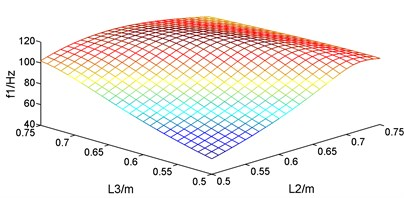a) The 1st natural frequency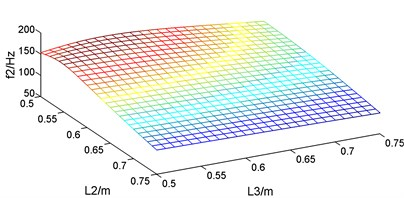b) The 2nd natural frequency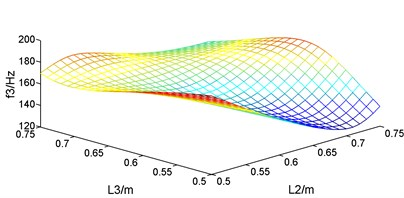c) The 3rd natural frequency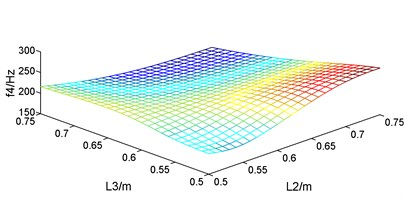d) The 4th natural frequency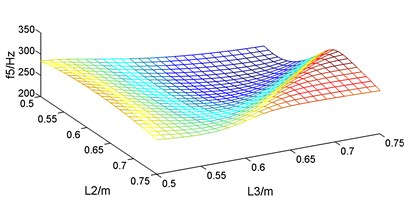e) The 5th natural frequency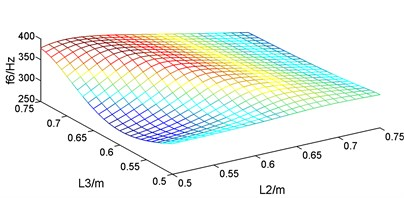f) The 6th natural frequency

For the vibration of the guide bar, first six modes account for most of the vibration energy, so the impact of the supports location on the first six natural frequencies is studied. According to the constraints condition, guide bar is symmetric with the total length as well as the length of the two cantilever sections fixed, so when ${L}_{2}$ and ${L}_{3}$ changes from 0.5 m to 0.75 m, ${L}_{4}$ changes from 0.625 m to 0.25 m. Let ${L}_{2}$ and ${L}_{3}$ changes by 0.01 m, and the first six corresponding natural frequencies are plotted as Fig. 5. It can be known from Fig. 5 that the first six natural frequencies changes with the supports location within constraint conditions, but the change law is different. So, the supports location where first six natural frequencies reached the maximum and the minimum are different, too.

The supports location where the natural frequencies reach the maximum and the minimum were calculated, as showed in Table 3. It can be known from Table 3 that when the length of segment ${L}_{2}=$ 0.50 m and ${L}_{3}=$ 0.50 m, the 1st natural frequency of guide bar ${f}_{1}=$ 54.8289 Hz; when ${L}_{2}=$ 0.66 m and ${L}_{3}=$ 0.64 m, the 1st natural frequency of guide bar ${f}_{1}$ researches to 116.4218 Hz, increasing the natural frequency by 2.12 times. When designing the guide bar shogging system of a warp knitting machine, the 1st natural frequency should be designed higher than the motor speed in order to avoid resonance. So, length of segment ${L}_{2}$ and ${L}_{3}$ should be designed around 0.65 m.

Table 3The supports location where the natural frequencies reach the maximum and the minimum

 The 1st natural frequency The 2nd natural frequency The 3rd natural frequency ${f}_{1max}$ / Hz ${f}_{1min}$ / Hz ${f}_{2max}$ / Hz ${f}_{2min}$ / Hz ${f}_{3max}$ / Hz ${f}_{3min}$ / Hz 116.4218 54.8289 164.8049 98.8352 192.4979 126.2099 ${L}_{2}$ / m 0.66 0.50 0.55 0.75 0.50 0.50 ${L}_{3}$ / m 0.64 0.50 0.52 0.75 0.50 0.71 The 4th natural frequency The 5th natural frequency The 6th natural frequency ${f}_{4max}$ / Hz ${f}_{4min}$ / Hz ${f}_{5max}$ / Hz ${f}_{5min}$ / Hz ${f}_{6max}$ / Hz ${f}_{6min}$ / Hz 263.2423 165.9190 327.3817 218.5197 386.9852 284.1712 ${L}_{2}$ / m 0.50 0.75 0.75 0.72 0.72 0.60 ${L}_{3}$ / m 0.74 0.75 0.66 0.54 0.54 0.50

### 5.2. The impact of support location on mode shapes

Guide bar is made of homogenous material and has uniform section. When there is great difference in vibration amplitude among segments, bending stresses concentrates at the large-amplitude segments and cause breakage while less stress exists in small-amplitude segments and hinder the exploiting of their performance. Usually, for avoiding the fracture of the large-amplitude segments, guide bar with larger section is used, not only wasting materials, but also increasing motor response time and energy consumption.

Let ${L}_{2}$ and ${L}_{3}$ changes by 0.01 m under the constraint conditions, the corresponding mode shapes at each location are plotted in Fig. 6, where $x$ is the axial coordination of the guide bar defined by each method, and u is the amplitude (by normalization processing, taking the max amplitude as 1). It can be known from the curve that each mode in different location has similar shape but different amplitude distribution. The short segments tend to have small amplitude while long segments have large amplitude. Only when the length of each segment is approximately equal can the bending deformation be homogeneous, avoiding the stress concentrate phenomenon caused by local large amplitude. Therefore, the length of each segments should be designed roughly equal when arranging supports locations. In this case, the length of segment ${L}_{2}$ and ${L}_{3}$ should be designed around 0.65 m.

## 6. Conclusions

1) Two methods (equivalent force method and segmental mode assuming method) of calculating the natural frequencies and mode shapes of a free-free-multi-supported beam subjected to an axial load is found, considering the structure characteristic of the guide bar, which has long length but small section, supported by many bearings, and subjected to an axial load. The results prove that these two methods are convenient for computer programing and have the same results in obtaining the natural frequencies and mode shapes of a free-free-multi-supported beam subjected to an axial load, solving the problem that the vibration function of this kind of beam cannot be simplified with the boundary condition of two ends.

Fig. 6First six mode shapes change with the supports location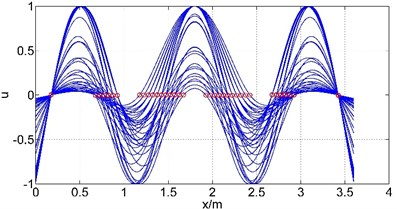a) The 1st natural frequency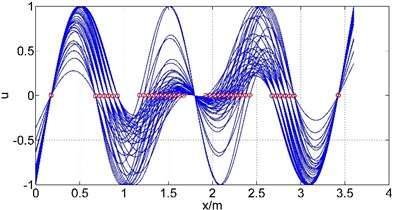b) The 2nd natural frequency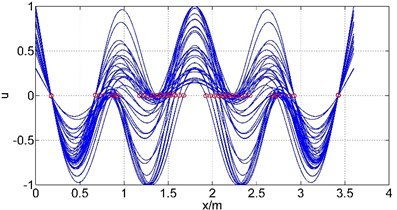c) The 3rd natural frequency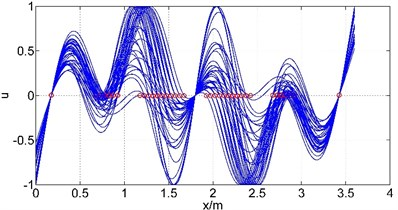d) The 4th natural frequency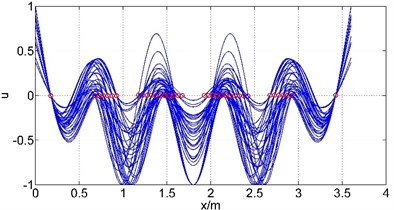e) The 5th natural frequency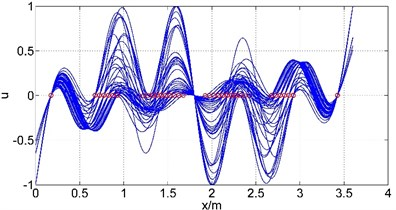f) The 6th natural frequency

2) The segmental mode assuming method is used to analyze the impact of the support location on the natural frequencies and mode shapes of the guide bar. The graphs of the change of natural frequencies with support locations are calculated, as well as the support locations where the natural frequencies reached the maximum and the minimum, providing a reference for the support location selection for the guide bar.

3) The curves of the change of the mode shapes with support location are plotted, which show that the short segment has small amplitude while long segment has large amplitude. Only when the length of each segment is approximately equal can the bending deformation be homogeneous, avoiding the phenomenon that bending stresses concentrates at the large-amplitude segments and cause breakage while less stress exists in small-amplitude segments and hinder the exploiting of their performance, providing a reference for the structure design of the guide bar.

07 June 2016
Accepted
19 September 2016
Published
15 May 2017
SUBJECTS
Mechanical vibrations and applications
Keywords
guide bar
supports location effect
vibration analysis
equivalent force method
segmental mode assuming method
Acknowledgements

This project is supported by National Natural Science Foundation of China (51375084) and Research Fund for the Doctoral Program of Higher Education of China (20130075110002).